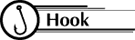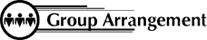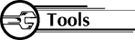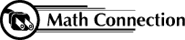8th Grade Math Home > Teacher Resources > Activities > Hands-On Equations: Student Worksheet

 Hands-On Equations: Student WorksheetUsing manipulatives allows students to better visualize mathematical concepts. The Hand-On Equations manipulatives help students "balance" the scale and "solve" equations. Students need to remember the property of equality, "whatever you do on one side of the equation, you must do to the other side"Students work individually or in pairsHands-On Equations ManipulativesEach student receives the Hands-On Equations kit. Review the property of equality, "whatever you do on one side of the equals sign, you must do on the other side." Review how to make zero sets. Goal: to get the variable on one side of the equation by itself. Demonstrate examples: x = 5 0 = 0, then -2 + 2 = 0 x + 3 = 5 2x = x - 2 3x - 1 = x + 5 Have students demonstrate 3x - 1 = x + 5. Hand out the Hands-On worksheet. Hands-On Equations Worksheet Use your Hands-On Equations kits to find the value of x. Then, check your answer on your paper. x – 3 = 1 x = ________ check: 2x = x + 5 x = ________ check: x + 6 = 2x – 3 x = ________ check: x – 2 = - 2x + 1 x = ________ check: 2(x + 1) = x + 9 x = ________ check:As a result of this activity, students can visualize the equality principle and will be able to have a better understanding of the steps used in solving equations.

 8th Grade Math Home > Teacher Resources > Activities > Hands-On Equations: Student Worksheet